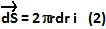## Monday, March 22, 2010

### Irodov Problem 3.19The electric flux through the circle is given by,Where E is the electric field and dS is the area vector through which E passes. From problem 3.14 we already know that E is given by,Consider an infinitesimally thin ring section of radius r and width dr. The area vector of this infinitesimally thin ring section is given by,Hence the flux is given by,